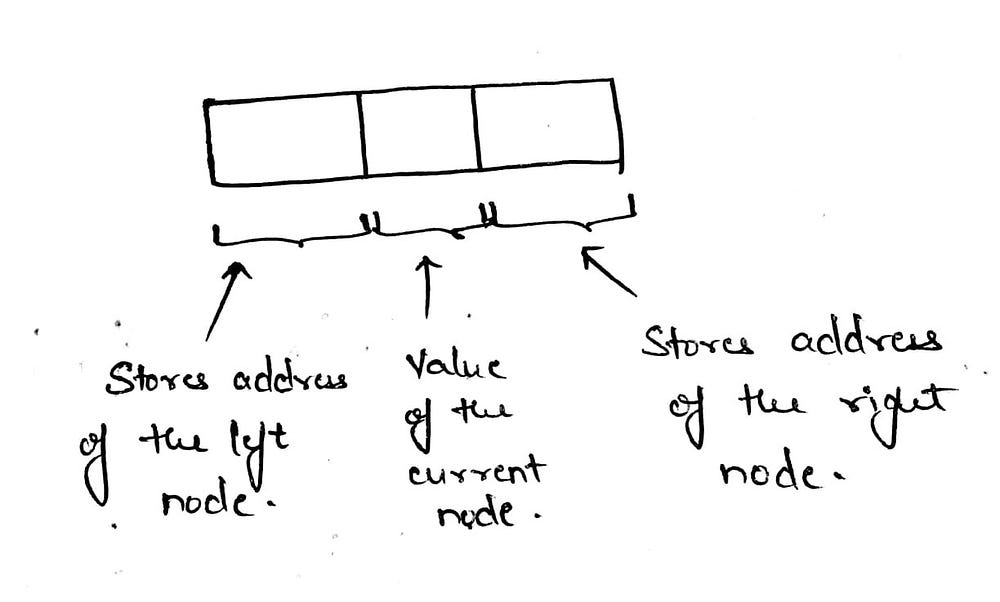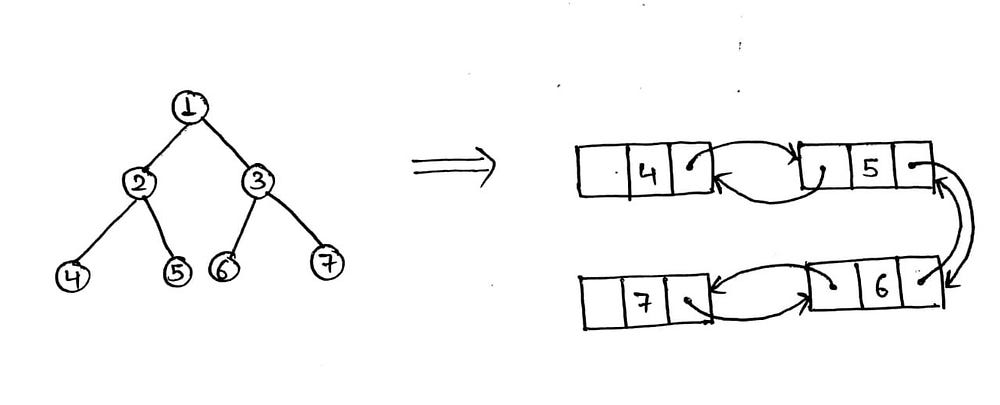Given a Binary Tree and the reference to the root node of the Tree, transform the leaf nodes of the Tree to a Doubly Linked List in Inorder sequence.

This problem can be broken down into parts — Find the leaf nodes of a Binary Tree and Given a set of elements, form a doubly linked list using them.

Find the leaf nodes of a Binary Tree -

To traverse a Binary Tree, we can Traverse the tree using Preorder, Inorder or Postorder Traversal Algorithms.

Preorder Traversal — ROOT -> LEFT -> RIGHT

Inorder Traversal — LEFT -> ROOT -> RIGHT

Postorder TRaversal — LEFT -> RIGHT -> ROOT

The solution to the current problem uses Inorder Traversal.

The algorithm for Inorder Traversal is as follows:

1. Input the root node.
2. If the root (current) node has no left or right child, then it is a leaf node, else goto Step(3)
3. If the root node is NULL then return else goto Step (4).
4. Read the root node of the left subtree and goto Step (1).
5. Print the value of the current node.
6. Read the root node of the right subtree and goto Step (2).

Given a set of elements, form a doubly linked list using them -

The Data Structure for the node of a doubly linked list is -A doubly linked list is different from a singly linked list because in a doubly linked list we can traverse in both the ways, from left to right and from right to left, whereas in a singly linked list, we can only traverse in one direction, either from left to right or from right to left.

We can form a doubly linked list from the given elements, by linked nodes with each other by iterating till the last node and linking the current node with the last node of the current linked list.The below given code is in C programming language.

```#include<stdio.h>
#include<stdlib.h>

struct node{
struct node *left;
int data;
struct node *right;
};

void leavesToDll(struct node *leaf){
struct node *first;
}
else{
while(first -> right != NULL){
first = first -> right;
}
first -> right = leaf;
leaf -> left = first;
}
}

void inorderTraversal(struct node *root){
if(root -> left == NULL && root -> right == NULL){
leavesToDll(root);
}
else if(root == NULL){
return;
}
else{
inorderTraversal(root -> left);
inorderTraversal(root -> right);
}
}

struct node *newNode(int data){
struct node *n = (struct node *)malloc(sizeof(struct node *));
n -> data = data;
n -> left = NULL;
n -> right = NULL;
return n;
}

void main(){
struct node *n = newNode(1);
n -> left = newNode(2);
n -> left -> left = newNode(4);
n -> left -> right = newNode(5);

n -> right = newNode(3);
n -> right -> left = newNode(6);
n -> right -> right = newNode(7);

inorderTraversal(n);